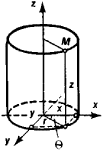# Cylindrical Coordinates

Also found in: Dictionary, Wikipedia.
Related to Cylindrical Coordinates: Spherical coordinates

## cylindrical coordinates

[sə′lin·drə·kəl ‚kō′ȯrd·ən·əts]
(mathematics)
A system of curvilinear coordinates in which the position of a point in space is determined by its perpendicular distance from a given line, its distance from a selected reference plane perpendicular to this line, and its angular distance from a selected reference line when projected onto this plane.
McGraw-Hill Dictionary of Scientific & Technical Terms, 6E, Copyright © 2003 by The McGraw-Hill Companies, Inc.
The following article is from The Great Soviet Encyclopedia (1979). It might be outdated or ideologically biased.

## Cylindrical Coordinates

The cylindrical coordinates of a point M are the three numbers r, θ, and z characterizing the position of M in space (see Figure 1). The coordinates are called cylindrical because the coordinate surface r = const is a cylinderFigure 1

whose elements are parallel to the z-axis. The relation between the cylindrical coordinates and the rectangular coordinates x, y, and z of M is given by the equations x = r cos θ, y = r sin θ, and z = z.

The Great Soviet Encyclopedia, 3rd Edition (1970-1979). © 2010 The Gale Group, Inc. All rights reserved.
References in periodicals archive ?
To calculate the four-group diffusion equation, we use the finite difference discretization of steady state four-group neutron diffusion equation in a cylindrical coordinate. First, the finite difference discretization of one group differential equation was established and later extended to four-group differential equation.
Due to the axial symmetry of the field, the magnetic moment vector [??] of the core under consideration has only an axial projection in cylindrical coordinates
Based on Kirchhoff plate theory for cylindrical coordinates, see Appendix 2, the relation between applied electric potential (V) and transverse displacement (w) is as follows:
In many applications, such as EM ground wave propagation from a lightning channel, a cylindrical coordinate is preferred.
Once we assume [g.sub.00] = [g.sub.11] = 1, we can switch to cylindrical coordinates {[rho], [phi], z, t}, by
To do it, persons in images are represented by a cylindrical model, and cylindrical coordinates of local features are then used in computing similarity between local features.
The purpose of this study is to develop a new clustering method for data in cylindrical coordinates based on the k-means.
We assume monochromatic field (with [e.sup.-i[omega]t] time dependence) and proceed with the analysis in circular cylindrical coordinate system to formulate Green's function of the problem and hence calculate the field distribution around the body in the plane of source.
Similar approaches have been used in  for the three-dimensional analysis of plates in rectilinear orthogonal coordinates and in  for an exact, three-dimensional, free vibration analysis of angle-ply laminated cylinders in cylindrical coordinates. The equations of motion written in orthogonal curvilinear coordinates allow general exact solutions for simply supported plate and shell geometries with constant radii of curvature.
where (r, [theta]) are cylindrical coordinates. Since x = r cos [theta] - b and y = r sin [theta], we find the torque as
To study the heat transfer between the eccentric cylinders, the energy equation is first written in bipolar cylindrical coordinates (21).

Site: Follow: Share:
Open / Close